# Wave Effect

The Wave effect adds a motion to the Z co-ordinate of the Object Mesh.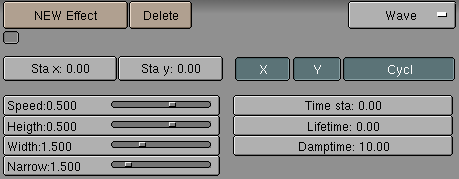Figure 29. Wave Control Panel

The wave effect influence is generated from a given starting point defined by the Sta X and Sta Y NumButs. These co-ordinates are in the Mesh local reference (Figure 30).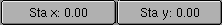Figure 30. Wave Origin

The Wave effect deformation originates from the given starting point and propagates along the Mesh with circular wavefronts, or with rectilinear wavefronts, parallel to the X or Y axis. This is controlled by two X and Y toggle buttons. If just one button is pressed fronts are linear, if both are pressed fronts are circular (Figure 31).

The wave itself is a gaussian-like ripple which can be either a single pulse or a series of ripples, if the Cycl button is pressed.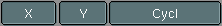Figure 31. Wave front type

The Wave is governed by two series of controls, the first defining the Wave form, the second the effect duration.

For what concerns Wave Form, controls are Speed, Height, Width and Narrow (Figure 32).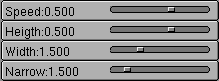Figure 32. Wave front controls

The Speed Slider controls the speed, in Units per Frame, of the ripple.

The Height Slider controls the height, in Blender Units and along Z, of the ripple (Figure 33).

If the Cycl button is pressed, the Width Slider states the distance, in Blender Units, between the topmost part of two subsequent ripples, and the total Wave effect is given by the envelope of all the single pulses (Figure 33).

This has an indirect effect on the ripple amplitude. Being ripples gaussian in shape, if the pulses are too next to each other the envelope could not reach the z=0 quote any more. If this is the case Blender actually lowers the whole wave so that the minimum is zero and, consequently, the maximum is lower than the expected amplitude value, as shown in Figure 33 at the bottom.

The actual width of each gaussian-like pulse is controlled by the Narrow Slider, the higher the value the narrower the pulse. The actual width of the area in which the single pulse is significantly non-zero in Blender Units is given by 4 over the Narrow Value. That is, if Narrow is 1 the pulse is 4 Units wide, and if Narrow is 4 the pulse is 1 Unit Wide.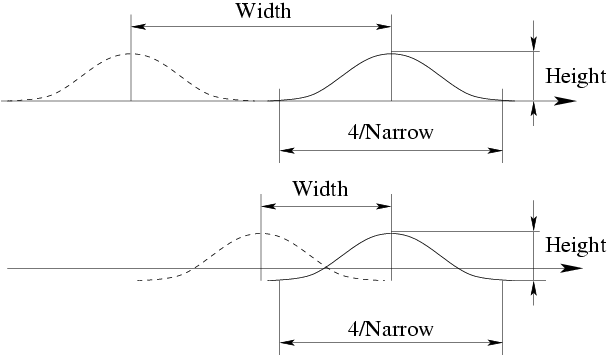Figure 33. Wave front characteristics

 To obtain a Sinusoidal-like waveTo obtain a nice Wave effect similar to sea waves and close to a sinusoidal wave it is necessary that the distance between following ripples and the ripple width are equal, that is the "Width" Slider value must be equal to 4 over the "Narrow" Slider value.

The last Wave controls are the time controls. The three NumButs define:

Time sta the Frame at which the Wave begins;

Lifetime the number of frames in which the effect lasts;

Damptime is an additional number of frames in which the wave slowly dampens from the Amplitude value to zero. The Dampening occurs for all the ripples and begins in the first frame after the "Lifetime" is over. Ripples disappear over "Damptime" frames.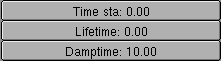Figure 34. Wave time controls